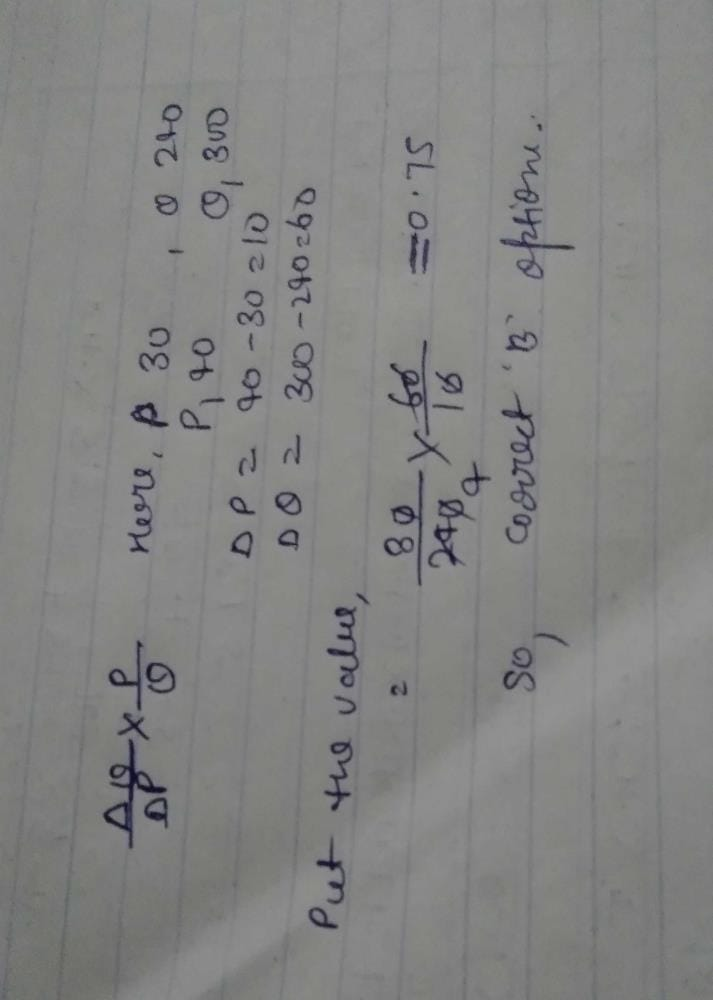If the price of apples rises from Rs. 30 per ...

### Related TestIf the price of apples rises from Rs. 30 per kg to Rs. 40 per kg and the supply increases from 240 kg to Rs. 300 kg. Elasticity of supply is

• a)
0.75

• b)
0.77

• c)
-0.67

• d)
-0.77MERAT RAM ASHOKArc Ed = [(Qd2 – Qd1) / midpoint Qd] ÷ [(P2 – P1) / midpoint P]

= [(300 – 240) / 270] ÷ [(40 – 30) / 35]

= 0.222/0.285

= 0.77

 1 Crore+ students have signed up on EduRev. Have you?Supriya Verma
If the price of apples rises from Rs. 30 per kg to Rs. 40 per kg and the supply increases from 240 kg to Rs. 300 kg. Elasticity of supply isa)0.75b)0.77c)-0.67d)-0.77Correct answer is option 'B'. Can you explain this answer?View courses related to this question Explore CA Foundation courses
 Explore CA Foundation coursesView courses related to this questionPriya Prithi
When question asks to find out elasticity of supply on given point ,then

we'll use general formula

as here the question wants us to find elasticity of supply between two prices

so using arc method formula is correct

• ### Suppose the price of movies seen at a theatre rises from rs120 per person t... more(Scan QR code)